Latest Teaching jobs   »   Lines, Angles And Triangle: Download Mathematics...

# Lines, Angles And Triangle: Download Mathematics Study Notes Free PDF For REET Exam

`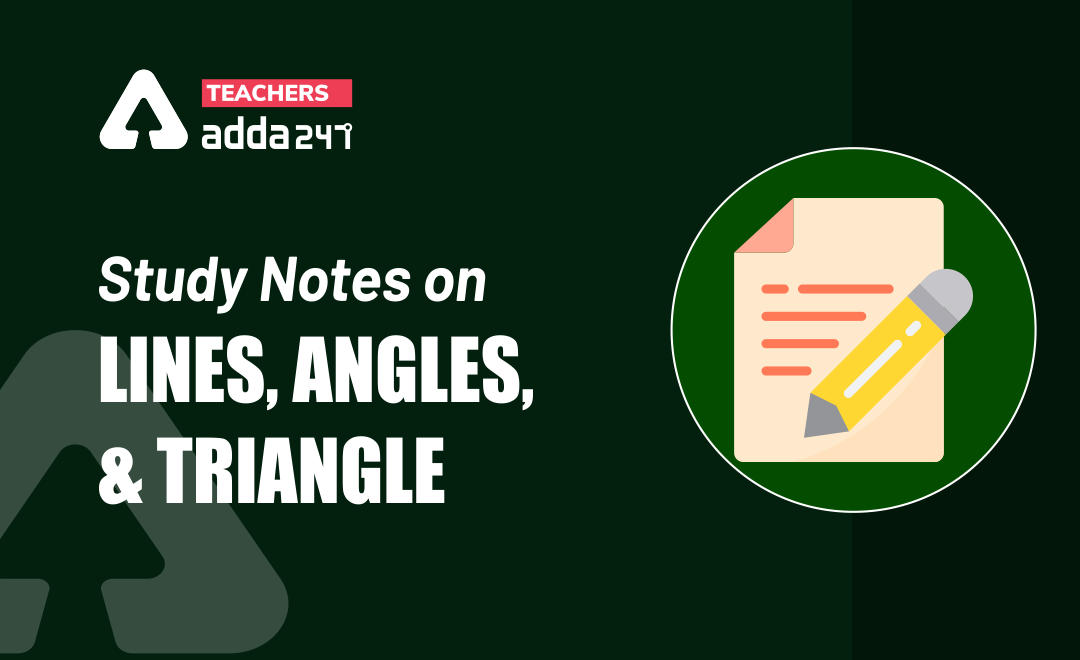`

Mathematics is an equally important section for  REETMPTET & DSSSB Exams and has even more abundant importance in some other exams conducted by central or state govt. Generally, there are questions asked related to basic concepts and properties of the Geometry.

To let you make the most of Mathematics section, we are providing important facts related to the Geometry.At least 2-3 questions are asked from geometry topic in most of the teaching exams. We wish you all the best of luck to come over the fear of the Mathematics section.

How to Overcome Exam Fever, Especially When You Fear Maths

## Geometry

Polygon: Two types of angle

1. Exterior
2. Interior
1. Exterior Angle: Sum of exterior Angle of the polygon is 360°,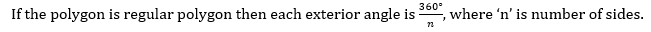Interior Angle: Sum of the interior angle of the polygon is (n – 2)× 180.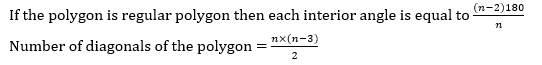1. Vertical opposite angle always be same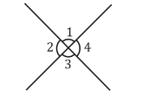-: ∠ 1 = ∠ 3  and ∠ 1 + ∠ 2= 180°

-: ∠ 2 = ∠ 4  and   ∠ 3 + ∠ 4 = 180°

Mathematics Study Notes For All Teaching Exams

1. Corresponding angles:

∠ 4 + ∠ 5 = 180°

∠ 3 + ∠ 6 = 180°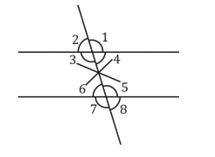1. Sum of 2 interior angle opposite to exterior angle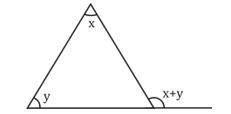5. In the given fig. AB = AC, then AD which is median of the triangle also be height of triangle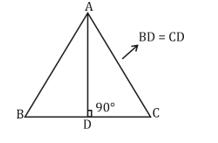• In the given fig. ABCD is a cyclic quadrilateral.∠ A + ∠ C = 180°  (opp. Angle)

∠ B + ∠ D = 180°

⟹ opposite interior angle is equal to exterior angle.

Get free Study material for REET Exam

• Centres of the triangle:

Type of centres:

• Centroid
• Incentre
• Circum-centre
• Ortho – centre

(1) Centroid: Intersecting points of the medians of triangle is known as centroid of the triangle.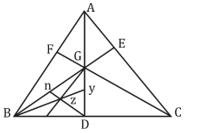Area of ∆ ABD = ∆ ACD

AG : GD = 2 : 1

Area of ∆ BGC = ∆ AGC = ∆ AGB

Area of ∆ nzGY : ∆ ABC

2 : 36

1 : 18

Example: PS is the median of a triangle PQR and O is centroid such that PS = 27 cm. The length of PO is

Sol. PS is the median and O is the centroid —– (given)

PS = 27 cm

Ratio of PO : OS

Practice REET Subject wise Quiz

(2) Incentre:  Intersecting points of angle bisector of triangle is known as Incentre of the triangle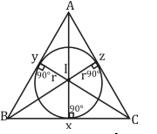Ix = Iy = Iz = radius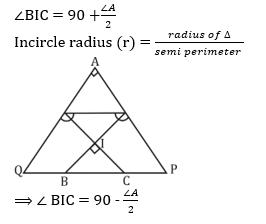Example: O is the incentre of triangle PQR, ∠ PQR = 70° and ∠PRQ = 60°, Then find the value of ∠ QOR.

Sol. Acc. to Question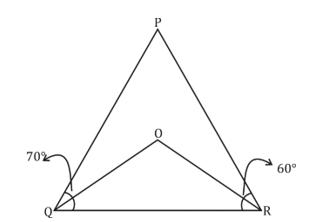QO and RO are the angle bisector

∴ ∠ RQO = 35° and ∠ QRO = 30°

In ∆ QOR, ∠ RQO + ∠ QRO + ∠ QOR = 180°

35° + 30° + ∠ QOR = 180°

∠ QOR = 180° – 65° ⟹ 115°

(3) Circum-centre: Intersecting point of the perpendicular bisector of triangle is known as circum-centre of the triangle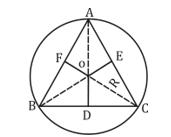AO = OB = OC = Radius

∠BOC = 2 (∠BAC)

In right ∠ ∆ circum-centre is formed on the mid-point of hypotenuse.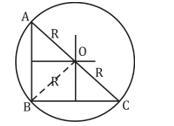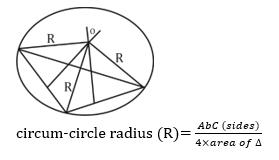(4) Ortho-centre: intersecting points of the altitudes of triangle is known as orthocentre of the triangle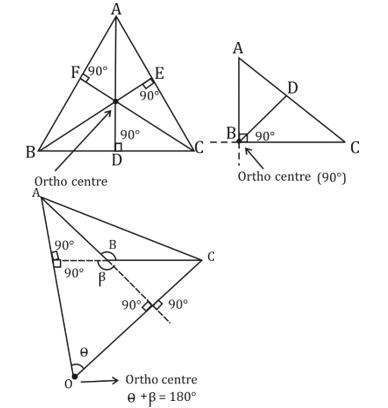Example:  In an obtuse angled triangle ABC, ∠B is obtuse angled and O is orthocentre. ∠ AOC = 69° and ∠ ABC is

Sol. ∠ ABC = 180° – ∠ AOC

= 180° – 69°

= 111°

Some important facts of the triangle:

1. Mid-Point Theorem: In triangle ABC, P and Q are mid – point of AB and AC. Then PQ always || to BC (PQ || BC).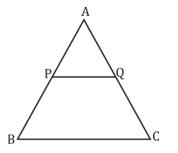1. Median theorem: In ∆ ABC, AD is Median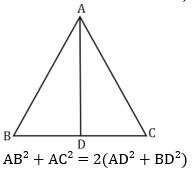Example: If the length of the three sides of a triangle is a 9 cm, 40 cm, and 41 cm then find the length of median to its greatest side.

Sol. This is a right-angled triangle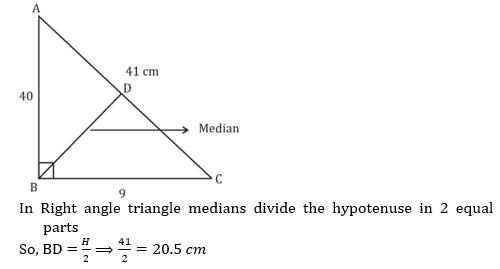• Angle bisector theorem: Internal angle bisector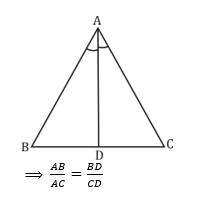External angle bisector: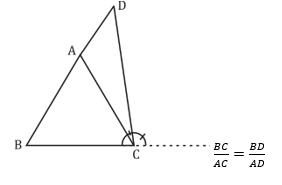• In the right Triangle ABC, F and D is the mid – points of AB and BC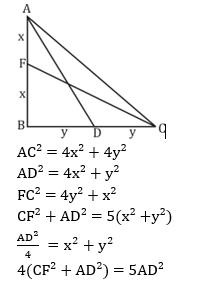### Download Geometry Study Notes PDF (Hindi)

Sharing is caring!

Thank You, Your details have been submitted we will get back to you.
•रस - परिभाषा, भे�...
•Learning Disabilities : Math Dyslexia, D...
•Flander's Interaction Category System (F...
•Kothari Commission (1964-66) कोठ�...
•विशेषण- परिभाष�...
•Phases of Teaching - Stages of Teaching ...